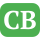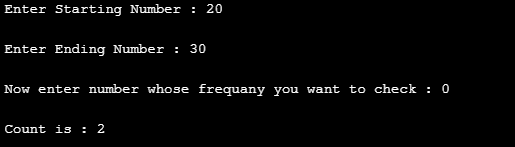CppBuzz.com

# Find frequency of any number between two numbers

This program ask user to input two numbers and one number whose frequency is to be calculated. Output of this code is number of times the number is present. Like 1 is present 2 times in 1 to 10

``````#include <stdio.h>

int main()
{
int startNo, endNo, no, counter, i;
counter = i = 0;

printf("%d", 123%10);

printf("\nEnter Starting Number : ");
scanf("%d", &startNo);
printf("\nEnter Ending Number : ");
scanf("%d",&endNo);

printf("\nNow enter number whose frequany you want to check : ");
scanf("%d",&no);

for(int i=startNo; i <=endNo; i++){
int temp = i;
while(temp!=0){
if(temp%10 == no)
counter++;
temp = temp/10;
}
}

printf("\nCount is : %d", counter);

return 0;
}
``````